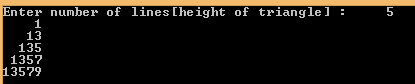## Saturday, 17 January 2015

### C Program to print a Right Angled Triangle

This is a simple C Program which prints out a Right angled triangle with respect to he input given.Right angled triangle

PROGRAM :

```#include <stdio.h>
#include <stdlib.h>

int main()
{
int i, j, n,k;
printf("Enter number of lines[height of triangle] : \t");
scanf("%d",&n);
for(i=1; i<=n; i++)
{
for(j=i; j<n; j++)
{
printf(" ");
}
for(k=1; k<(i*2); k++)
{

printf("%d",k++);
}
printf("\n");
}
return 0;
}```

OUTPUT :C Program to print  Right angled triangle

NOTE : The output should be less than 5 to see the best result as when the output exceeds 5 the numbers from 10,11 .. are 2 digits so then the output would become somewhat messy.